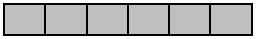# Fraction Operations

## Objective

Decompose non-unit fractions and represent them as a whole number times a unit fraction.

## Common Core Standards

### Core Standards

?

• 4.NF.B.4.A — Understand a fraction a/b as a multiple of 1/b. For example, use a visual fraction model to represent 5/4 as the product 5 × (1/4), recording the conclusion by the equation 5/4 = 5 × (1/4).

?

• 3.NF.A.1

• 3.NF.A.2

## Criteria for Success

?

1. Understand that repeated addition expressions can be more efficiently represented using multiplication and use that understanding to transition from decompositions of fractions as a sum of unit fractions to a multiple of unit fractions.
2. Decompose a fraction less than or equal to 1 into a multiple of unit fractions, recording the decomposition with an equation.
3. Justify decompositions with a visual model, such as a tape diagram or number line.

#### Fishtank Plus

• Problem Set
• Student Handout Editor
• Vocabulary Package

?

### Problem 1

1. Use the first equation below to fill in the blanks for the second equation.

a.   ${2+2+2+2+2=5}$ groups of $2$

b.   ${{1\over4}+{1\over4}+{1\over4}=}$ ____ groups of ____

2. Replace the right side of the equations above with a numerical expression.

### Problem 2

Decompose the fraction represented below into a sum of unit fractions and a multiple of the unit fraction. Record the decompositions with equations.### Problem 3

Decompose the following fractions into a multiple of unit fractions. Write an equation to record your decomposition.

a.   ${{4\over5}}$

b.   ${{12\over12}}$

## Discussion of Problem Set

?

• What is an advantage to representing the fractions using multiplication?
• What is similar in Problems 3(c), 3(d), and 3(e)? What is different?
• Are you surprised to see multiplication equations with products less than 1? Why?
• How is multiplying fractions like multiplying whole numbers?

?

Decompose the following fraction as a sum of unit fractions as well as a multiple of unit fractions. Record the decompositions with equations.

${{6\over9}}$

#### References

EngageNY Mathematics Grade 4 Mathematics > Module 5 > Topic A > Lesson 3Exit Ticket

Grade 4 Mathematics > Module 5 > Topic A > Lesson 3 of the New York State Common Core Mathematics Curriculum from EngageNY and Great Minds. © 2015 Great Minds. Licensed by EngageNY of the New York State Education Department under the CC BY-NC-SA 3.0 US license. Accessed Dec. 2, 2016, 5:15 p.m..

Modified by The Match Foundation, Inc.

?